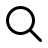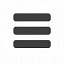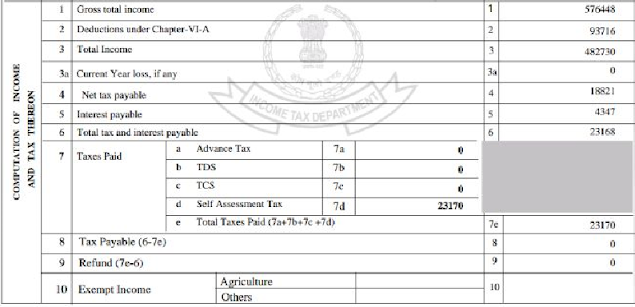# Knowledge Fusion- A Preparation Guide to Achieve|IBPS SBI RRB SSC exams

Daily Preparation Material & Quizzes for General Awareness,Computer,Banking,GS and many more...### Accounts Banking Balance Sheet 19 ratio analysis explanation || CAIIB JAIIB TipsEdge.com

Bank Balance sheet Ratio analysis JAIIB and CAIIB

Ratio analysis is one of the most important section in jaiib. It covers almost 10 marks.
Knowledge of ratio analysis is necessary to understand the financial position of a company.
There is a standard ratio for each ratio ( CURRENT RATIO it is 2:1 ).
There are a total of 19 ratios you need to know to get 10marks.

1.       CURRENT RATIO
2.       ACID TEST RATIO/ QUICK RATIO
3.       Fixed asset turnover ratio
4.       Current asset turnover ratio
5.       WORKING CAPITAL TURNOVER RATIO
6.       DEBTORS TURNOVER RATIO
7.       CREDITORS TURNOVER RATIO
8.       STOCK TURNOVER RATIO
9.       DEBT EQUITY
10.   TOTAL ASSET TO DEBT RATIO
11.   PROPRIETARY RATIO
12.   INTEREST COVERAGE RATIO
13.   GROSS PROFIT RATIO
14.   NET PROFIT RATIO
15.   OPERATING PROFIT RATIO
16.   OPERATING RATIO
17.   RETURN ON CAPITAL EMPLOYED
18.   EARNING PER SHARE
19.   PRICE EARNING RATIO

Let's first understand the terms present in the balance sheet and their classification. You should learn all the balance sheet terms what they mean exactly. I will explain to you all the terms that come in the balance sheet. In order to solve these ratios, you need to understand the simple balance sheet terms like (land and building is a FIXED ASSET). This is the list of items that appear on the balance sheet.

Let's understand each of them one by one. It is simple to learn this way.

1) Current Assets – Current assets are those which can be converted to cash within a year.
·         Cash in hand
·         Cash at bank
·         Sundry debtors
·         Closing stock
·         Short term investment·         Stock
·         Tax provision
·         Receivables
·         Debtors
·         Income Accrued         Prepaid expences
·         Cash equivalents
Note: Quick asset=current assets-stock and prepaid expenses

2)Current Liabilities – Those liabilities to be paid within 1 year
·         Creditors
·         Sundry creditors
·         Bank Over Draft/ Cash credit
·         Bills payable
·         Outstanding expenses/ expenses payable
·         Provisions
·         Provision for tax
·         Dividend
Note :
Quick liability= Current liabilities —bank overdraft
Quick asset=current assets-stock and prepaid expenses
Working capital = Current Assets- Current Liabilities
Turnover = sales

20.   CURRENT RATIO = Current assets / current liabilities

21.   ACID TEST RATIO or QUICK RATIO = quick assets / quick liabilities

3) Quick asset :  Current  asset – stock – prepaid expences
·         Cash in hand
·         Debtors
4) Quick liabilities: Current liabilities – bank Overdraft/cash credit

5)Networth
·         Reserves
·         Capital
6)Long term liability
·         Term loan
·         Debentures
7)Fixed asset
·         Land and building
·         Machinery
·

In the question, there will be given current liabilities current assets capital fixed assets etc we have to organize and follow the total of 19  formulas in ratio analysis JAIIB OR CAIIB EXAM  out of which 6 are turned over ratios. Remember turnover means “SALES

22.   Fixed asset turnover ratio
§  = sales/net fixed asset

23.   Current asset turnover ratio
§  =sales/net current asset

24.   WORKING CAPITAL TURNOVER RATIO
§  =sales / working capital
§  Working capital = current assets – current liabilities

25.   DEBTORS TURNOVER RATIO
§  =Net credit sales/avg accounts receivables
§  =(op debtors + clo debtors)/2+BR
§  Note: credit sale=total sale-cash sale
§  Average collection period=365/debtors turnover ratio

26.   CREDITORA TURNOVER RATIO
§  =Net credit purchase / average accounts payables
§  Net Credit purchase=total purchase-purchased return –cash purchase
§  Avg accounts payable=creditors+ bills payable  ([OPENING CREDITOR+CLOSING CREDITOR)/2 ,( OPENING BILLS PAYABLE + CLOSING BILLS PAYABLE )/2]
§  Avg payment period=365 days /creditors turn over ratio

27.   STOCK TURNOVER RATIO
§  =sales / avg stock  (avg stock=opening stock+closing stock /2)
§  Cost of goods sold=opening stock +purchases-closing stock+direct expenses

The income tax of a company with the financial position assessment is given in the following image.

28.   GROSS PROFIT RATIO
§  Profit depends on sales cost of goods sold
§  =sales-cogs
§  =Gross profit/net sales*100
§  From the above figure gross profit is 576448
§  To get operating profit è 576448- (office and administration expenses)
§  Operating profit is the income before interest and tax paid=482730
§  Total tax paid here is 23170
§  Net profit = operating profit (482730) – total tax paid(23170) =459560
§  If a company has equity and dividend
§  Then retained earning = net profit –equity and dividend

The order of income statement follows as
Gross profit à operating profit à profit before interest and tax à profit after interest before tax
àprofit after inter taxes and pref dividend à retained earning à

problems will be solved soon

On the balance sheet liabilities side you need to classify 3 items(LTL,NW,CL) and in assets side 5 items(CA,FA,NCA,IA,CA-QA). will be posted soon

<-------------------***------------------->
Thank You
<-------------------***------------------->